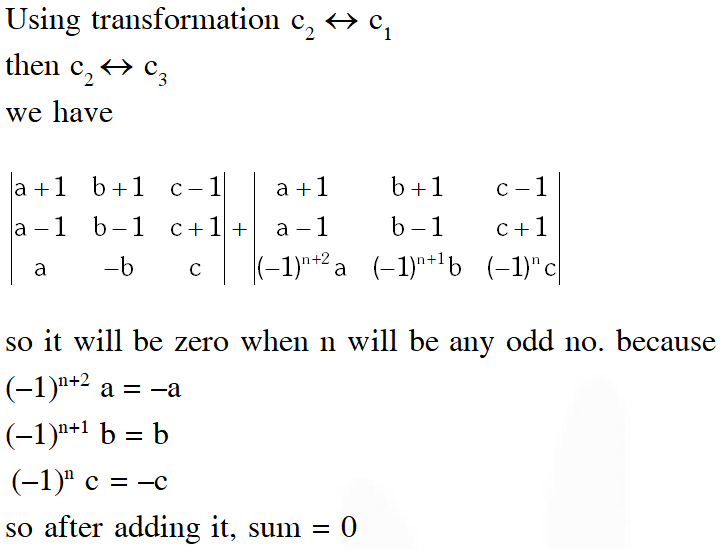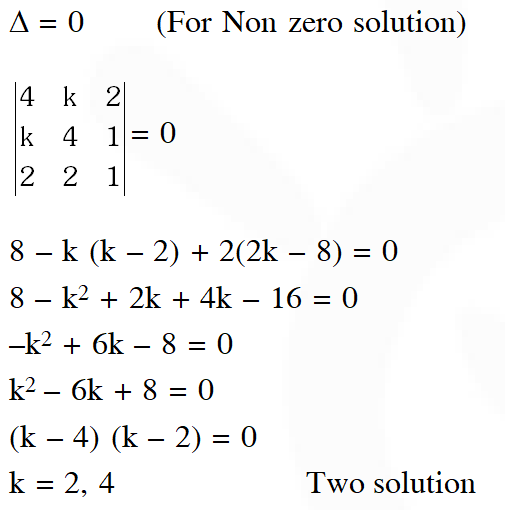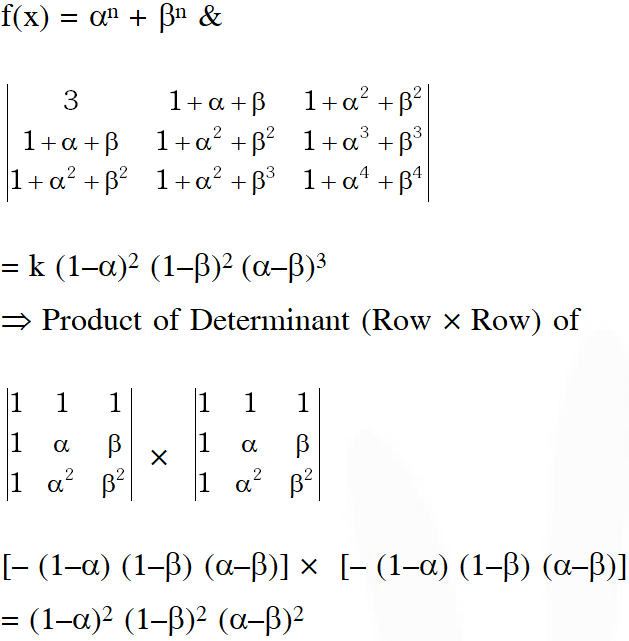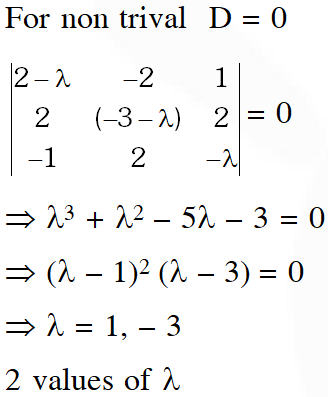Download the eSaral app and start learning from Kota's top IITians and doctors.

# Determinant - JEE Main Previous Year Question with Solutions

JEE Main Previous Year Question of Math with Solutions are available at eSaral. Practicing JEE Main Previous Year Papers Questions of mathematics will help the JEE aspirants in realizing the question pattern as well as help in analyzing weak & strong areas. eSaral helps the students in clearing and understanding each topic in a better way. eSaral is providing complete chapter-wise notes of Class 11th and 12th both for all subjects. Besides this, eSaral also offers NCERT Solutions, Previous year questions for JEE Main and Advance, Practice questions, Test Series for JEE Main, JEE Advanced and NEET, Important questions of Physics, Chemistry, Math, and Biology and many more. Download eSaral app for free study material and video tutorials.
Q. Let $a, b, c$ be such that $\mathrm{b}(\mathrm{a}+\mathrm{c}) \neq 0 . \mathrm{If}$ If $\left|\begin{array}{ccc}{a} & {a+1} & {a-1} \\ {-b} & {b+1} & {b-1} \\ {c} & {c-1} & {c+1}\end{array}\right|+\left|\begin{array}{ccc}{a+1} & {b+1} & {c-1} \\ {a-1} & {b-1} & {c+1} \\ {-1} & {a} & {(-1)^{n+1} b} & {(-1)^{n} c}\end{array}\right|=0$ then the value of n is :- (1) Any odd integer (2) Any integer (3) Zero (4) Any even integer [AIEEE - 2009]
Ans. (1)Q. Consider the system of linear equations : $\mathrm{x}_{1}+2 \mathrm{x}_{2}+\mathrm{x}_{3}=3$ $2 \mathrm{x}_{1}+3 \mathrm{x}_{2}+\mathrm{x}_{3}=3$ $3 x_{1}+5 x_{2}+2 x_{3}=1$ The system has (1) Infinite number of solutions (2) Exactly 3 solutions (3) A unique solution (4) No solution [AIEEE - 2010]
Ans. (4) Here $D=0$ $\& \quad D_{1} \neq 0$ so we can say no solution
Q. The number of values of k for which the linear equations 4x + ky + 2z = 0 kx + 4y + z = 0 2x + 2y + z = 0 possess a non-zero solution is :- (1) 1 (2) zero (3) 3 (4) 2 [AIEEE - 2011]
Ans. (4)Q. If the trivial solution is the only solution of the system of equations x – ky + z = 0 kx + 3y – kz = 0 3x + y – z = 0 Then the set of all values of k is: (1) {2, –3}      (2) R – {2, –3}     (3) R – {2} (4) R – {–3} [AIEEE - 2011]
Ans. (2) Here for trival solution $D \neq 0$ So $\mathrm{D}=\left|\begin{array}{ccc}{1} & {-\mathrm{k}} & {1} \\ {\mathrm{k}} & {3} & {-\mathrm{k}} \\ {3} & {1} & {-1}\end{array}\right|=0$ $\Rightarrow \mathrm{D}=2 \mathrm{k}^{2}-12+2 \mathrm{k}=0 \Rightarrow \mathrm{k}=-3,2$ so $\mathrm{R}-\{-3,2\}$
Q. The number of values of k, for which the system of equations : (k + 1)x + 8y = 4k kx + (k + 3)y = 3k – 1 has no solution, is – (1) infinite (2) 1 (3) 2 (4) 3 [JEE(Main)-2013]
Ans. (2) $\frac{k+1}{k}=\frac{8}{k+3}=\frac{4 k}{3 k-1}$ (1) = (2) $\Rightarrow \quad k^{2}-4 k+3=0$ k = 1, 3 for k = 1 (2) = (3) for $\mathrm{k}=3 \quad(2) \neq(3)$ k = 3
Q. If $\alpha, \beta \neq 0,$ and $f(\mathrm{n})=\alpha^{\mathrm{n}}+\beta^{\mathrm{n}}$ and $\left|\begin{array}{ccc}{3} & {1+f(1)} & {1+f(2)} \\ {1+f(1)} & {1+f(2)} & {1+f(3)} \\ {1+f(2)} & {1+f(3)} & {1+f(4)}\end{array}\right|=\mathrm{K}(1-\alpha)^{2}(1-\beta)^{2}(\alpha-\beta)^{2}$ then K is equal to : (1) $\alpha \beta$ (2) $\frac{1}{\alpha \beta}$ (3) 1 (4) –1 [JEE(Main)-2014]
Ans. (3)$\therefore k=1$
Q. The set of all values of $\lambda$ for which the system of linear equations : $2 \mathrm{x}_{1}-2 \mathrm{x}_{2}+\mathrm{x}_{3}=\lambda \mathrm{x}_{1}$ $2 \mathrm{x}_{1}-3 \mathrm{x}_{2}+2 \mathrm{x}_{3}=\lambda \mathrm{x}_{2}$ $-\mathrm{x}_{1}+2 \mathrm{x}_{2}=\lambda \mathrm{x}_{3}$ has a non-trivial solution (1) contains two elements (2) contains more than two elements (3) is an empty set (4) is a singleton [JEE(Main)-2015]
Ans. (1)Q. The system of linear equations $\mathrm{x}+\lambda \mathrm{y}-\mathrm{z}=0$ $\lambda \mathrm{x}-\mathrm{y}-\mathrm{z}=0$ $\mathrm{x}+\mathrm{y}-\lambda \mathrm{z}=0$ has a non-trivial solution for : (1) exactly three values of $\lambda$ (2) infinitely many values of $\lambda$ (3) exactly one value of $\lambda$ (4) exactly two values of $\lambda$ [JEE(Main)-2016]
Ans. (1) $\left|\begin{array}{ccc}{1} & {\lambda} & {-1} \\ {\lambda} & {-1} & {-1} \\ {1} & {1} & {-\lambda}\end{array}\right|=0 \quad \Rightarrow \quad \lambda=0,1,-1$
Q. If S is the set of distinct values of 'b' for which the following system of linear equations x + y + z = 1 x + ay + z = 1 ax + by + z = 0 has no solution, then S is : (1) a singleton (2) an empty set (3) an infinite set (4) a finite set containing two or more elements [JEE(Main)-2017]
Ans. (1) $D=\left|\begin{array}{lll}{1} & {1} & {1} \\ {1} & {a} & {1} \\ {a} & {b} & {1}\end{array}\right|=0 \Rightarrow a=1$ and at a = 1 $\mathrm{D}_{1}=\mathrm{D}_{2}=\mathrm{D}_{3}=0$ But at a = 1 and b = 1 $\left.\begin{array}{ll}{\text { First two equations are }} & {x+y+z=1} \\ {\text { and third equation is }} & {x+y+z=0}\end{array}\right] \Rightarrow$ There is nosolution. $\mathrm{b}=\{1\} \Rightarrow$ it is a singleton set
Q. If $\left|\begin{array}{ccc}{x-4} & {2 x} & {2 x} \\ {2 x} & {x-4} & {2 x} \\ {2 x} & {2 x} & {x-4}\end{array}\right|=(A+B x)(x-A)^{2},$ then the ordered pair $(A, B)$ is equal to : (1) (–4, 3)            (2) (–4, 5)             (3) (4, 5)               (4) (–4, –5) [JEE(Main)-2018]
Ans. (2) $\left|\begin{array}{ccc}{\mathrm{x}-4} & {2 \mathrm{x}} & {2 \mathrm{x}} \\ {2 \mathrm{x}} & {\mathrm{x}-4} & {2 \mathrm{x}} \\ {2 \mathrm{x}} & {2 \mathrm{x}} & {\mathrm{x}-4}\end{array}\right|=(\mathrm{A}+\mathrm{Bx})(\mathrm{x}-\mathrm{A})^{2}$ Put $x=0 \Rightarrow\left|\begin{array}{ccc}{-4} & {0} & {0} \\ {0} & {-4} & {0} \\ {0} & {0} & {-4}\end{array}\right|=A^{3} \Rightarrow A=-4$ $\left|\begin{array}{ccc}{\mathrm{x}-4} & {2 \mathrm{x}} & {2 \mathrm{x}} \\ {2 \mathrm{x}} & {\mathrm{x}-4} & {2 \mathrm{x}} \\ {2 \mathrm{x}} & {2 \mathrm{x}} & {\mathrm{x}-4}\end{array}\right|=(\mathrm{Bx}-4)(\mathrm{x}+4)^{2}$ $\left|\begin{array}{ccc}{1-\frac{4}{\mathrm{x}}} & {2} & {2} \\ {2} & {1-\frac{4}{\mathrm{x}}} & {2} \\ {2} & {2} & {1-\frac{4}{\mathrm{x}}}\end{array}\right|=\left(\mathrm{B}-\frac{4}{\mathrm{x}}\right)\left(1+\frac{4}{\mathrm{x}}\right)^{2}$ Put $\mathrm{x} \rightarrow \infty \quad \Rightarrow \quad\left|\begin{array}{lll}{1} & {2} & {2} \\ {2} & {1} & {2} \\ {2} & {2} & {1}\end{array}\right|=\mathrm{B} \Rightarrow \mathrm{B}=5$ ordered pair (A, B) is (–4, 5)
Q. If the system of linear equations $x+k y+3 z=0$ $3 x+k y-2 z=0$ $2 x+4 y-3 z=0$ has a non-zero solution $(\mathrm{x}, \mathrm{y}, \mathrm{z}),$ then $\frac{\mathrm{xz}}{\mathrm{y}^{2}}$ is equal to : (1) 10 (2) – 30 (3) 30 (4) –10 [JEE(Main)-2018]
Ans. (1)
Q. If the system of linear equations : $x+a y+z=3$ $\mathrm{x}+2 \mathrm{y}+2 \mathrm{z}=6$ $x+5 y+3 z=b$ has no solution, then :- (1) $a=-1, b=9$ (2) $a \neq-1, b=9$ (3) $a=1, b \neq 9$ (4) $a=-1, b \neq 9$ [JEE(Main)-2018]
Ans. (4)
Q. The number of values of k for which the system of linear equations, (k+2)x + 10y = k kx + (k+3) y = k – 1 has no solution is : (1) infinitely many (2) 1 (3) 2 (4) 3 [JEE(Main)-2018]
Ans. (2)

May 20, 2021, 8:32 a.m.
Kitni mistakes ha thik kro Shame on
Ihwfhwofzxofg
Feb. 25, 2021, 10:07 a.m.
Zihfsogzzoajzf
Mohd bilal
Feb. 18, 2021, 9:13 p.m.